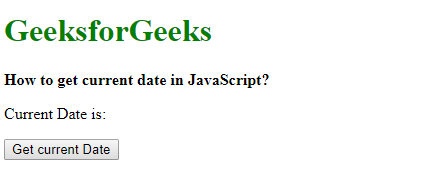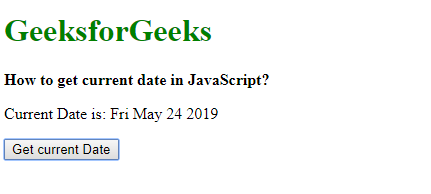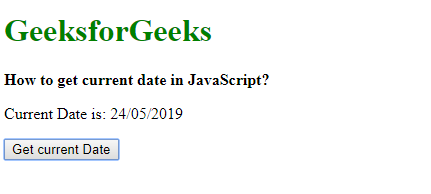# How to get the current date in JavaScript ?

Method 1: Using Date().toDateString() method: The toDateString() method is used to return only the date part of a Date object. The first three letters specify the day of the week, the next three letters specify the month name, the next two digits specify the day of the month and the last four digit year specify the year.
This method returns a String containing the date in the above format. This is a better method than slicing for the date portion in the Date() object.

Syntax:

`new Date().toDateString()`

Example:

 ` ` `<``html``> ` `     `  `<``head``> ` `    ``<``title``> ` `        ``How to get current date in JavaScript? ` `    `` ` ` ` ` `  `<``body``> ` `    ``<``h1` `style``=``"color: green"``> ` `        ``GeeksforGeeks ` `    `` ` `     `  `    ``<``b``> ` `        ``How to get current date in JavaScript? ` `    `` ` `     `  `    ``<``p``> ` `        ``Current Date is:  ` `        ``<``span` `class``=``"output"``> ` `    `` ` ` `  `    ``<``button` `onclick``=``"getCurrentDate()"``> ` `        ``Get current Date ` `    `` ` `     `  `    ``<``script` `type``=``"text/javascript"``> ` `        ``function getCurrentDate() { ` `            ``let date = new Date().toDateString(); ` ` `  `            ``document.querySelector('.output').textContent ` `                    ``= date; ` `        ``} ` `    `` ` ` ` ` `  `                     `

Output:

• After clicking the button:• After clicking the button:Method 2: Getting the day, month and year using their respective methods: All the parts of a date can be found by their respective methods. The day can be extracted by using the getDate() method, the month can be extracted by using the getMonth() method and the year can be extracted by using the getFullYear() method. The day and month are padded with 0s if they are single digits by converting it to a String object and using the padStart() method. This method pads the current string until the resulting string reaches the given length, here it is padded to a length of 2. The final date is then constructed by concatenating all these parts in the format as required.

Syntax:

```    let dateObj = new Date();
let month = String(dateObj.getMonth() + 1).padStart(2, '0');
let year = dateObj.getFullYear();
let output = day + '/' + month + '/' + year;
```

Example:

 ` ` `<``html``> ` ` `  `<``head``> ` `    ``<``title``> ` `        ``How to get current date in JavaScript? ` `    `` ` ` ` ` `  `<``body``> ` `    ``<``h1` `style``=``"color: green"``> ` `        ``GeeksforGeeks ` `    `` ` `     `  `    ``<``b``> ` `        ``How to get current date in JavaScript? ` `    `` ` `     `  `    ``<``p``> ` `        ``Current Date is:  ` `        ``<``span` `class``=``"output"``> ` `    `` ` ` `  `    ``<``button` `onclick``=``"getCurrentDate()"``> ` `        ``Get current Date ` `    `` ` `     `  `    ``<``script` `type``=``"text/javascript"``> ` `        ``function getCurrentDate() { ` ` `  `            ``let dateObj = new Date(); ` `            ``let month = String(dateObj.getMonth() + 1).padStart(2, '0'); ` `            ``let day = String(dateObj.getDate()).padStart(2, '0'); ` `            ``let year = dateObj.getFullYear(); ` `            ``let output = day + '/' + month + '/' + year; ` ` `  `            ``document.querySelector('.output').textContent = output; ` `        ``} ` `    `` ` ` ` ` `  `                     `

Output:

• Before clicking the button:• After clicking the button:My Personal Notes arrow_drop_upIm listening

If you like GeeksforGeeks and would like to contribute, you can also write an article using contribute.geeksforgeeks.org or mail your article to contribute@geeksforgeeks.org. See your article appearing on the GeeksforGeeks main page and help other Geeks.

Please Improve this article if you find anything incorrect by clicking on the "Improve Article" button below.